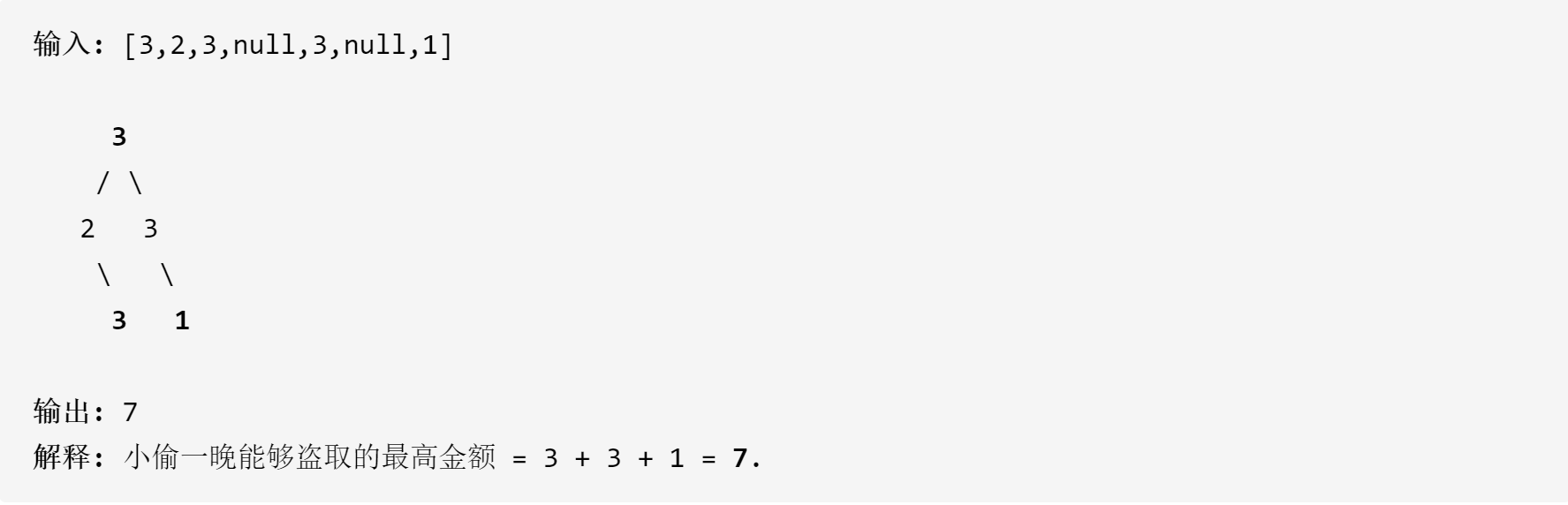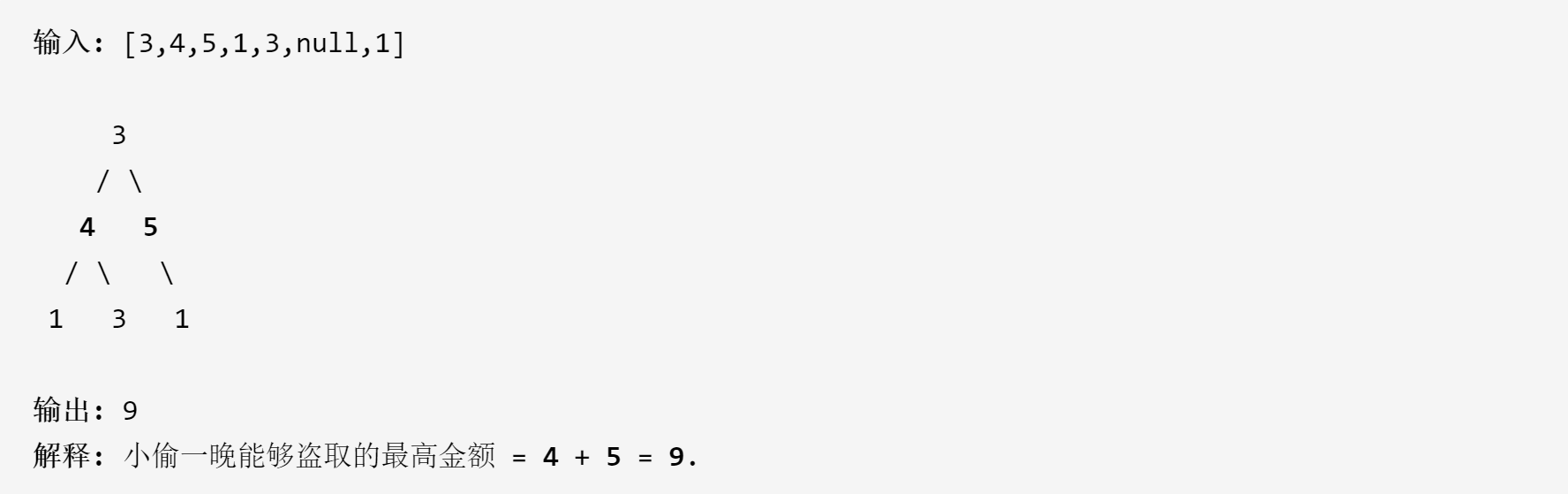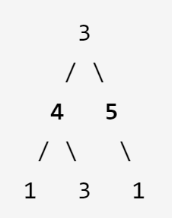## Leetcode day4:打家劫舍III_hit1180300517的博客-程序员秘密

#### 原题：

（话说这个小偷这么聪明为什么不去当程序员）``````/**
* Definition for a binary tree node.
* public class TreeNode {
*     int val;
*     TreeNode left;
*     TreeNode right;
*     TreeNode(int val) { this.val = val;
*  	}
*  }
* }
*/
``````

#### 思路和代码：

##### 层序遍历代码（与题意不符）：
`````` public int rob(TreeNode root) {

int odd = 0;
int even = 0;
int loft = 0;
int curLevel = 1;
int nextLevel = 0;
TreeNode now = root;
q.offer(now);
if(now==null)return 0;
while(!q.isEmpty()){

now = q.poll();
curLevel--;

if(loft%2==0) {

even += now.val;
}
else{

odd += now.val;
}

if(now.left!=null) {

q.offer(now.left);
nextLevel++;
}
if(now.right!=null) {

q.offer(now.right);
nextLevel++;
}
if(curLevel==0) {

curLevel = nextLevel;
nextLevel = 0;
loft++;
}
}

return Math.max(odd,even);
}
``````##### 简单递归代码：
``````class Solution {

public int rob(TreeNode root) {

if(root == null)return 0;
if(root.left == null&&root.right == null){

return root.val;
}
int left = 0,right = 0,valleft = 0,valright = 0;
left = rob(root.left);
right = rob(root.right);
if(root.left!=null) {

valleft = rob(root.left.left) + rob(root.left.right);
}
else valleft = 0;
if(root.right!=null) {

valright = rob(root.right.left) + rob(root.right.right);
}
else valright = 0;
return Math.max(root.val+valleft+valright,left+right);
}
}
``````

##### 动态规划代码：
``````public class Solution {

public int rob(TreeNode root) {

Map<TreeNode,Integer> m = new HashMap<>();
return new_rob(root,m);
}
int new_rob(TreeNode root,Map<TreeNode,Integer> m) {

int left = 0,right = 0,valleft = 0,valright = 0,result = 0;
if(root == null)return 0;
if(root.left == null&&root.right == null) {

return root.val;
}
if(m.containsKey(root)) {

return m.get(root);
}

right = new_rob(root.right,m);
left = new_rob(root.left,m);

if(root.right!=null) {

valright = new_rob(root.right.left,m) + new_rob(root.right.right,m);
}
else valright = 0;
if(root.left!=null) {

valleft = new_rob(root.left.left,m) + new_rob(root.left.right,m);
}
else valleft = 0;
result = Math.max(root.val+valleft+valright,left+right);
m.put(root, result);
return result;
}

}
``````

##### 优化思路：

（这一部分是借鉴大佬的）

root = Math.max(rob(root.left), rob(root.left)) + Math.max(rob(root.right), rob(root.right))
root = rob(root.left) + rob(root.right) + root.val;

``````public int rob(TreeNode root) {

int[] result = robInternal(root);
return Math.max(result, result);
}

public int[] robInternal(TreeNode root) {

if (root == null) return new int;
int[] result = new int;

int[] left = robInternal(root.left);
int[] right = robInternal(root.right);

result = Math.max(left, left) + Math.max(right, right);
result = left + right + root.val;

return result;
}

``````

### 基于依存句法分析的开放式中文实体关系抽取_CopperDong的博客-程序员秘密_开放式实体关系抽取

这一段时间一直在做知识图谱，卡在实体关系抽取这里几个月了，在github上面看到有人使用卷积神经网络训练模型进行抽取，自己也尝试了一下，但是一直苦于没有像样数据去训练，而标注训练集又太费时间了，我不太愿意干体力活。所以采用了一个低档次的方法，基于依存句法分析的实体关系抽取，记录一下心得，方便日后忘记可以再找回来。    本方法参考了github上面的项目和一篇论文，在文章末尾给出，使用的分词...

### tinyxml的使用以及示例_aa838260772的博客-程序员秘密

1.下载xml源代码：github上面 clone地址：https://github.com/aughey/tinyxml/2.下来以后自己make一下主要的就几个头文件和源文件3.测试案例4.编译成静态库： ar rv libxml.a  *.o5. 测试案例运行: g++ -o test test.o -L./ -lxml6.问题：对于main程序，先编译成目标文件，最